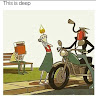2

# Four Keys Keyboard

Difficulty: MEDIUMContributed By
Priyaraj
Avg. time to solve
30 min
Success Rate
70%

Problem Statement
Suggest Edit

#### Imagine you have a special keyboard with the following four keys:

``````Key 1: (A): Print one ‘A’ on screen.
Key 2: (Ctrl-A): Select the whole screen.
Key 3: (Ctrl-C): Copy selection to buffer.
Key 4: (Ctrl-V): Print buffer on screen appending it after what has already been printed.
``````

#### Given a positive integer ‘N’, find out the maximum numbers of ‘A’s you can print on the screen if you are allowed to press the keys of this special keyboard ‘N’ times.

##### Input Format:
``````The first line of input contains an integer ‘T’ denoting the number of test cases.
The next ‘T’ lines represent the ‘T’ test cases.

The first and only line of each test case contains a positive integer ‘N’, representing the number of times you can press the keys of the keyboard.
``````
##### Output Format:
``````For each test case, print in a separate line, the maximum number of ‘A’s that you can print on the screen.
``````
##### Follow-up:
``````Try solving the problem in O(N) complexity.
``````
##### Note:
``````You do not need to print anything, it has already been taken care of. Just implement the given function.
``````
##### Constraints:
``````1 <= T <= 50
1 <= N <= 150

Where ‘T’ is the number of test cases and  ‘N’ is the integer representing the number of times you can press the keys of the keyboard.

Time Limit: 1 sec
``````
##### Sample Input 1:
``````2
3
7
``````
##### Sample Output 1:
``````3
9
``````
##### Explanation For Sample Input 1:
``````Test case 1:
We can at most get 3 A's on the  screen by pressing the following key sequence:
A, A, A

Test case 2:
We can at most get 9 A's on the screen by pressing the following key sequence:
A, A, A, Ctrl-A, Ctrl-C, Ctrl-V, Ctrl-V as mentioned below
Press Key(A), Screen will have “A”
Press Key(A) again, Screen will have “AA”
Press Key(A) again, Screen will have “AAA”
Press Key(Ctrl-A), This will select all three A’s which are printed on the screen.
Press Key(Ctrl-C), This will copy all three A’s in the buffer.
Press Key(Ctrl-V), It appends 3 ‘A’s from the buffer on screen, So the screen will now have “AAAAAA”.
Press Key(Ctrl-V), It appends 3 ‘A’s from the buffer on screen, So the screen will now have “AAAAAAAAA”.
``````
##### Sample Input 2:
``````2
11
1
``````
##### Sample Output 2:
``````27
1
``````
##### Explanation For Sample Input 2:
``````Test case 1:
We can at most get 27 A's on the screen by pressing the following key sequence.
A, A, A, Ctrl-A, Ctrl-C, Ctrl-V, Ctrl-V, Ctrl-A, Ctrl-C, Ctrl-V, Ctrl-V

Test case 2:
We can at most get 1 ‘A’ on the screen by pressing key ‘A’ once.
``````Console